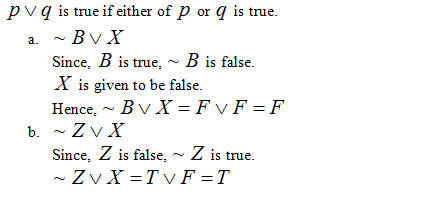# Compute the truth values of the following symbolic statements, supposing that the truth value of A, B, C is T, and the truth value of X, Y, Z is F. a. ~B ⋁ Xb. ~Z ⋁ X

Question
1 views

Compute the truth values of the following symbolic statements, supposing that the truth value of A, B, C is T, and the truth value of X, Y, Z is F.

a. ~B ⋁ X

b. ~Z ⋁ X

check_circle

Step 1 If p is some statement, then if p is true then ~p is false and vice versa....

### Want to see the full answer?

See Solution

#### Want to see this answer and more?

Solutions are written by subject experts who are available 24/7. Questions are typically answered within 1 hour.*

See Solution
*Response times may vary by subject and question.
Tagged in